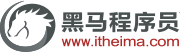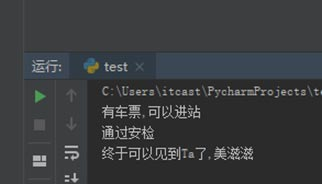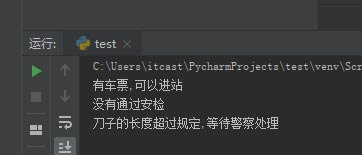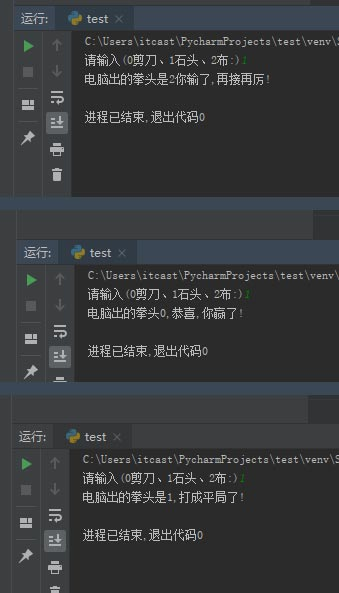# python中if嵌套的用法[python培训]if嵌套指的是在if或者if…else语句里面包含if或if-else语句，其嵌套的格式如下：

```if 条件1:
满足条件1做的事情1
满足条件1做的事情2
…(省略)…
if 条件2
满足条件2做的事情1
满足条件2做的事情2
…(省略)…```

```ticket = 1  # 用1代表有车票，0代表没有车票
Knife_length = 9    # 刀子的长度，单位为cm
if ticket == 1:
print("有车票，可以进站")
if Knife_length < 10:
print("通过安检")
print("终于可以见到Ta了，美滋滋")
else:
print("没有通过安检")
print("刀子的长度超过规定，等待警察处理")
else:print("没有车票，不能进站")
print("亲爱的，那就下次见了，一票难求啊~~~~")``````import random
player_input= input("请输入(0剪刀、1石头、2布:)")
player = int (player_input)
computer = random.randint(0， 2)
if (player ==0 and computer == 2) or (player ==1 and computer ==0) or (player == 2 and computer ==1):
print("电脑出的拳头%s，恭喜，你赢了!"%computer)
elif (player == 0 and computer == 0) or (player == 1 and computer == 1) or (player == 2 and computer== 2):
print("电脑出的拳头是%s，打成平局了!" %computer)
else:
print("电脑出的拳头是%s你输了，再接再厉!" %computer)```python+人工智能课程